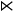# Maths - Notation

## Multidimensional Algebra

notation meaning basis Alternativedirect sum or Kronecker sum depending on contextdirect product or Kronecker product depending on context
/\ exterior algebrascalar or real depending on context (see below) e0² 2 dimensional vector e1,e2³ 3 dimensional vector e1,e2,e3n n dimensional vector e1…en² bivector based on 2D algebra e12³ bivector based on 3D algebra e12,e31,e23
C complex numbersH quaternions³
O octonions7
aA a is an element of the set A

## Scalar

I have used the term 'scalar' interchangeably with the term 'real', that is, a continuous value that can be represented by a single number.

Strictly speaking the term 'scalar' should be reserved for a quantity that is used to scale a vector, that is change its magnitude without changing its direction, or in other words a scalar is the ratio of parallel vectors.

For instance I should not really call energy a scalar because there are no vectors involved.

I apologise for my lack of mathematical rigor here, its just that the word scalar seems to better express that it is not a vector and its less likely to cause confusion with the real part of a complex number. Also this (mis?)usage is quite common in the computer world.

## Matrix

notation meaning
[M] matrix
[M]t transpose of matrix (swap rows & columns)
[M]-1 inverse of matrix
[I] identity matrix (ones on leading diagonal, otherwise zeros)

The individual elements of the matrix are numbered as follows,

 m00 m01 m02 … m0n m10 m11 m12 … m1n m20 m21 m22 … m2nmp0 mp1 mp2 … mpn

The first subscript represents the row, the second subscript represents the column

### Tensor Notation

notation example meaning pages in site
subscript e1 coordinate basis
superscript x1 coordinate value
Einstein Summation Convention eixi=e1x1+e2x2+e3x3=∑eixi When the same index appears twice in an expression, once raised and once lowered, a sum is implied.
partial derivatives a=∂/∂a

## Set Theory

notation example meaning
A A = {a | aA} set
a aA element of A
{a, b, c}   set containing a, b and c
a H = {ah | hH} left coset of a
(a,b)   cycle notation
<a,b>   all the elements of group generated by a and b
<r,f | r³=1, f²=1, frf=r-1> generators with constrains
 K
K = {k | kK} complement of set indicated by bar over top
| A |   grade (size) = number of elements in A
| G : H |   number of left cosets of H in G
×   Direct ProductSemidirect Product (left){n, h}φ {n',h'} Semidirect Product (right)Bicrossed Product
also known as Knit or Zappa-Szep producth = h'H such that
a h' = h a
Normal subgroup a H = H a for all a in G
Ø   empty set
¬   not
and (= intersection)
or (U = union)is in (is an element of the set)AB A is contained in B (or A is a subset of B)
->   if
<->   if and only iffor allthere existsis isomorphic to(S)   The set of subsets of S (power set)
orb(s)   Orbit - set of elements that can be reached
stab(s)   Stabilizer - set of elements that don't move s

### Definition

Endo is a Greek word meaning inside.

## Logic / Proofs

notation example meaning pages in site
/\

conjunction, and , intersection (in set), greatest lower bound (in order)

\/   disjunction, or , union (in set), least upper bound (in order)
T   Top (true)
_|_   Bottom (false)
=> A => B Implication
A => B means A implies BTφ

φ is a theorem of T
that is: φ is deducible from T

In contrast to 'implication' above this is a statement about statements usually written only when we mean φ follows from T, wheras A => B is a statement we may consider as a conjecture . See [Lawvere,Rosebrugh page 198]

Not to be confused with right adjoint in category theory.

logicfor all (quantifier)there exists (quantifier)

## Category Theory

notation example meaning pages in site
AB   Map / Morphism / Function between mathematical structures that relates or preserves the structures in some way. May be injection, surjection or bijection (injection can be specifically indicated see below)
ab   A function in terms of elements of the structures aA, bBA function that is valid if all the functions drawn as solid lines are validinjective morphism (embedding)canonical map - map of G onto factor group G/H where H is a normal subgroup of GLeft adjointRight adjoint

## Denotional Semantics

notation example meaning pages in site
[[D]] [[2*5]] = 10 A 'denotion' is written in double square brackets.
It represents the meaning of D to a program
evaluation of DBottom (undefined)Less defined than

## Greek Alphabet

upper lower   upper lower   upper lower
Alpha A α Iota I ι Rho P ρ
Beta B β Kappa K κ Sigma Σ σ
Gamma Γ γ Lambda Λ λ Tau Τ τ
Delta Δ δ Mu M μ Upsilon Υ υ
Epsilon E ε Nu N ν Phi Φ φ
Zeta Z ζ Xi Ξ ξ Chi X χ
Eta H η Omicron O ο Psi Ψ ψ
Theta Θ θ Pi Π π Omega Ω ω

## Various Operator Symbols

 × ÷ ± • ° ð Ð ƒ µ Ø ø Þ þ † … ∂ ◊ ≤ ≥ ∈ ≠ ≈ ∫ ∞ ∀ ∃ √

### Powers of 10

 m 10-3 K 103 µ 10-6 M 106 n 10-9 G 109 p 10-12 T 1012 f 10-15 a 10-18